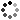# Halloween Middle School Math Project - Pumpkin Math

On Sale
\$4.00
\$4.00
Preview

Are you looking for an exciting way to engage your middle school math students this Halloween season? Look no further! Pumpkin math is a perfect middle school math Halloween activity that combines the magic of Halloween with the power of mathematics.

This activity is best for middle school math students who have the following skills.

Skills:

• Percent error calculation
• Create a histogram
• Understand outliers
• Calculate mean, median, mode and range
• Create and have a basic understanding of box-and-whisker plots.
• Calculate cost of multiple pumpkins given unit price.
• Calculate cost of time to carve pumpkins give unit rate.

Supplies:

• Pumpkins (you can do one per class, per group or per student)
• String and ruler (to measure circumference)
• Carving supplies
• Pumpkin Math packet

NOTE: If you have multiple classes then you can share the data between classes if you need more data.

You can also make up the seed count data if you’re not really into carving pumpkins. ;)

ACTIVITIES

Estimate and Percent Error: Students estimate the weight, circumference and seed count of a pumpkin. Students then determine the actual weight, circumference and seed count of a pumpkin and calculate their percent error.

Data Analysis: Students create and analyze a histogram of percent error (either of weight, circumference and seed count).

Real – World Problems: Students solve real-world pumpkin math problems. Students calculate cost of pumpkins given the unit cost. Students also determine the time to carve multiple pumpkins given the unit rate.

Seed Statistics: Students calculate the mean, median, mode and range of seed count collected from various pumpkins. Students will then create and analyze a box-and-whisker plot.

A data sheet is included as a way for students to record data collected from other students.

Time: If you choose to complete the whole packet, I would set aside 3 math periods for this activity. One for the pumpkin carving and two to gather data, complete the packet and have a class discussion if you choose.

Please feel free to contact me with any questions!

Michelle,

Make Sense of Math

One purchase is for one teacher/family. You may only use this item with students in a single classroom/family.